Скачать презентацию CHAPTER 6 Random Variables 6 2 Transforming and

f078914428c0475c555f8bde0f8f6907.ppt

• Количество слайдов: 11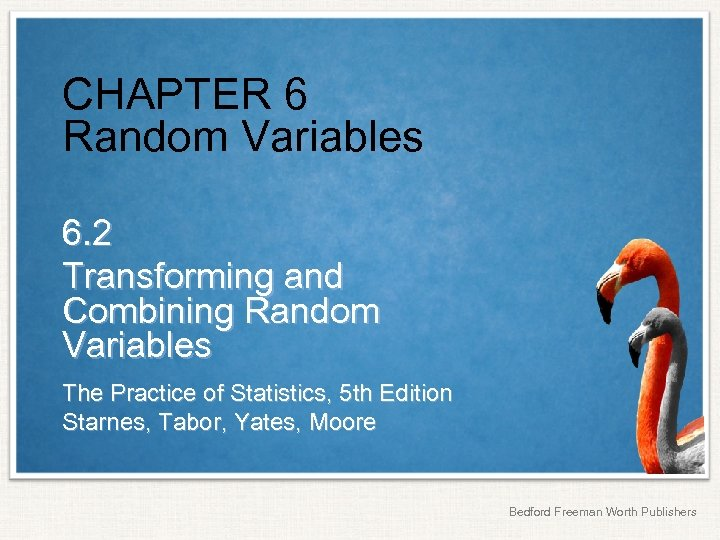CHAPTER 6 Random Variables 6. 2 Transforming and Combining Random Variables The Practice of Statistics, 5 th Edition Starnes, Tabor, Yates, Moore Bedford Freeman Worth Publishers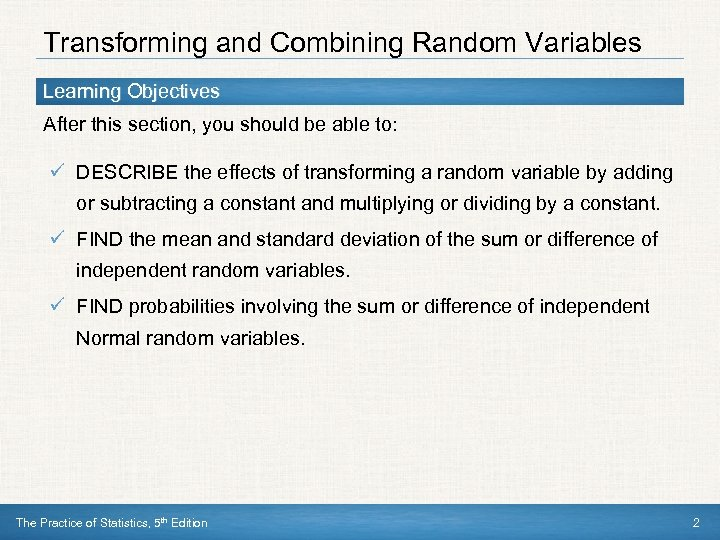Transforming and Combining Random Variables Learning Objectives After this section, you should be able to: ü DESCRIBE the effects of transforming a random variable by adding or subtracting a constant and multiplying or dividing by a constant. ü FIND the mean and standard deviation of the sum or difference of independent random variables. ü FIND probabilities involving the sum or difference of independent Normal random variables. The Practice of Statistics, 5 th Edition 2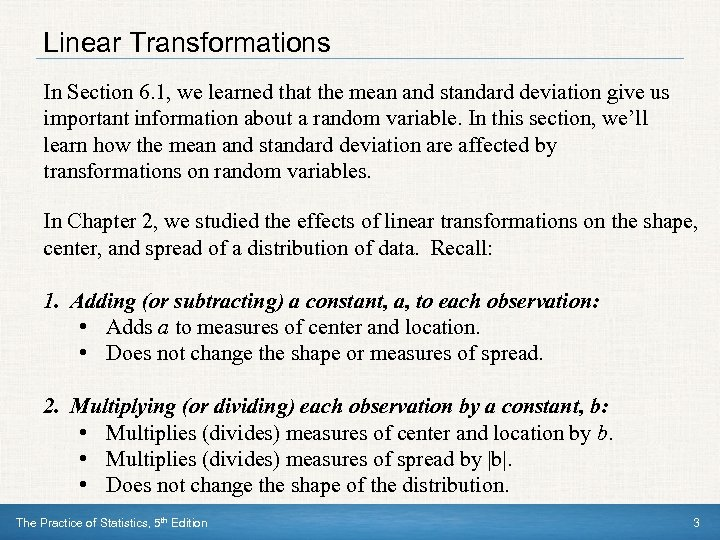Linear Transformations In Section 6. 1, we learned that the mean and standard deviation give us important information about a random variable. In this section, we’ll learn how the mean and standard deviation are affected by transformations on random variables. In Chapter 2, we studied the effects of linear transformations on the shape, center, and spread of a distribution of data. Recall: 1. Adding (or subtracting) a constant, a, to each observation: • Adds a to measures of center and location. • Does not change the shape or measures of spread. 2. Multiplying (or dividing) each observation by a constant, b: • Multiplies (divides) measures of center and location by b. • Multiplies (divides) measures of spread by |b|. • Does not change the shape of the distribution. The Practice of Statistics, 5 th Edition 3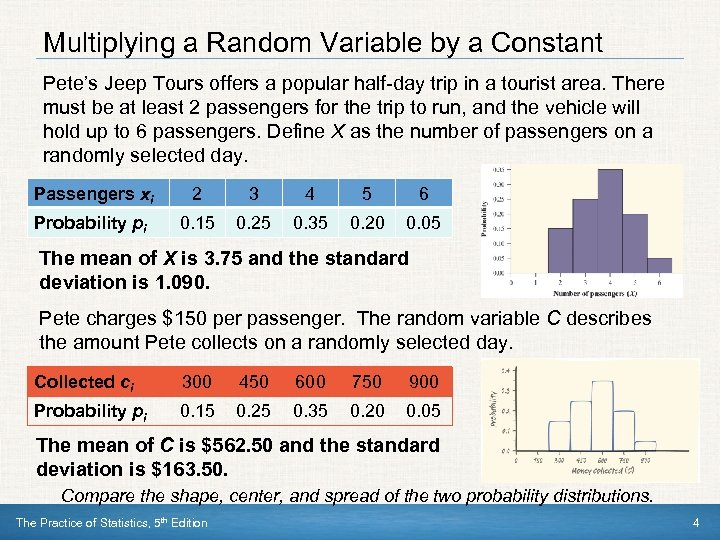Multiplying a Random Variable by a Constant Pete’s Jeep Tours offers a popular half-day trip in a tourist area. There must be at least 2 passengers for the trip to run, and the vehicle will hold up to 6 passengers. Define X as the number of passengers on a randomly selected day. Passengers xi 2 3 4 5 6 Probability pi 0. 15 0. 25 0. 35 0. 20 0. 05 The mean of X is 3. 75 and the standard deviation is 1. 090. Pete charges \$150 per passenger. The random variable C describes the amount Pete collects on a randomly selected day. Collected ci 300 450 600 750 900 Probability pi 0. 15 0. 25 0. 35 0. 20 0. 05 The mean of C is \$562. 50 and the standard deviation is \$163. 50. Compare the shape, center, and spread of the two probability distributions. The Practice of Statistics, 5 th Edition 4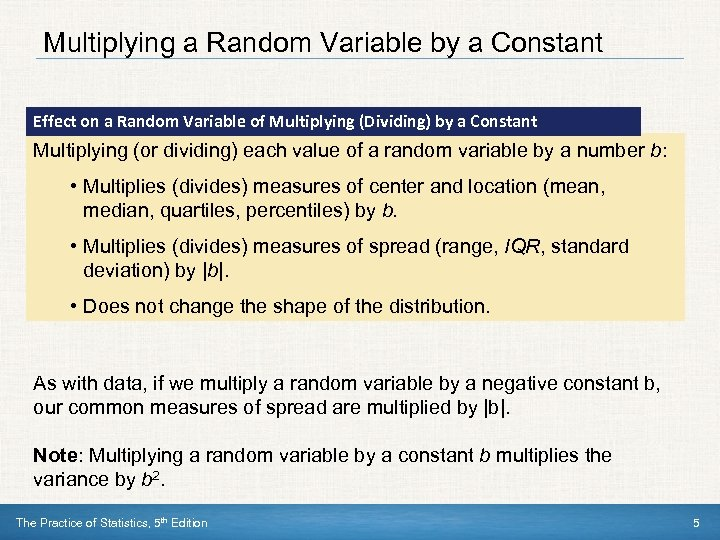Multiplying a Random Variable by a Constant Effect on a Random Variable of Multiplying (Dividing) by a Constant Multiplying (or dividing) each value of a random variable by a number b: • Multiplies (divides) measures of center and location (mean, median, quartiles, percentiles) by b. • Multiplies (divides) measures of spread (range, IQR, standard deviation) by |b|. • Does not change the shape of the distribution. As with data, if we multiply a random variable by a negative constant b, our common measures of spread are multiplied by |b|. Note: Multiplying a random variable by a constant b multiplies the variance by b 2. The Practice of Statistics, 5 th Edition 5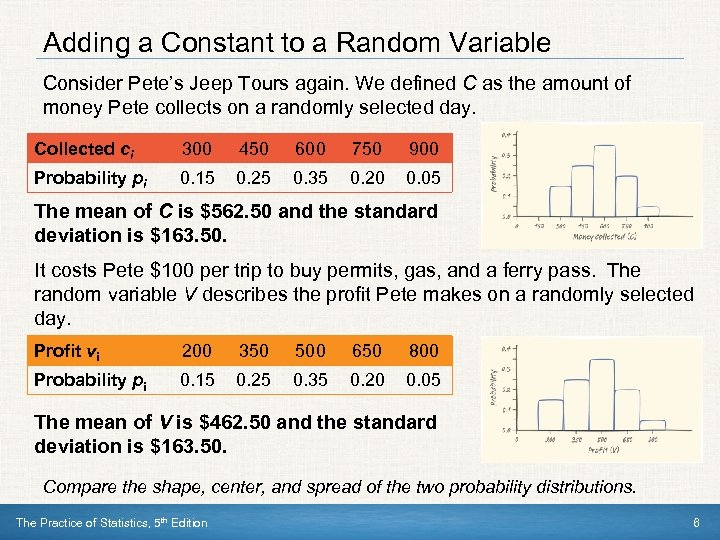Adding a Constant to a Random Variable Consider Pete’s Jeep Tours again. We defined C as the amount of money Pete collects on a randomly selected day. Collected ci 300 450 600 750 900 Probability pi 0. 15 0. 25 0. 35 0. 20 0. 05 The mean of C is \$562. 50 and the standard deviation is \$163. 50. It costs Pete \$100 per trip to buy permits, gas, and a ferry pass. The random variable V describes the profit Pete makes on a randomly selected day. Profit vi 200 350 500 650 800 Probability pi 0. 15 0. 25 0. 35 0. 20 0. 05 The mean of V is \$462. 50 and the standard deviation is \$163. 50. Compare the shape, center, and spread of the two probability distributions. The Practice of Statistics, 5 th Edition 6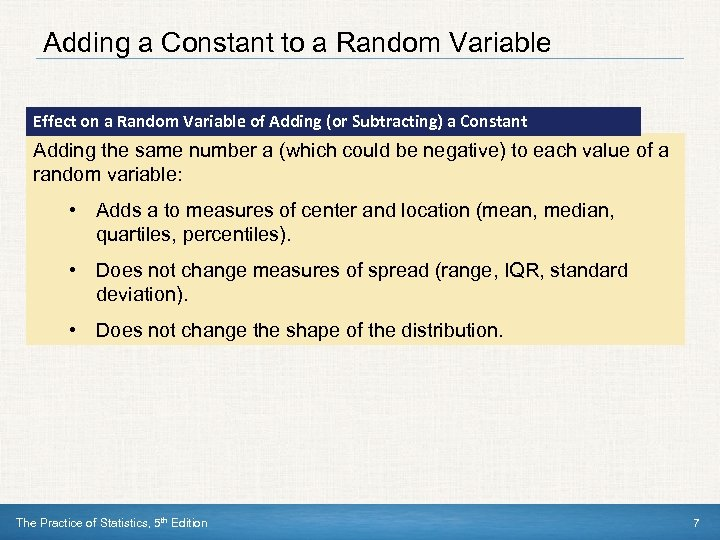Adding a Constant to a Random Variable Effect on a Random Variable of Adding (or Subtracting) a Constant Adding the same number a (which could be negative) to each value of a random variable: • Adds a to measures of center and location (mean, median, quartiles, percentiles). • Does not change measures of spread (range, IQR, standard deviation). • Does not change the shape of the distribution. The Practice of Statistics, 5 th Edition 7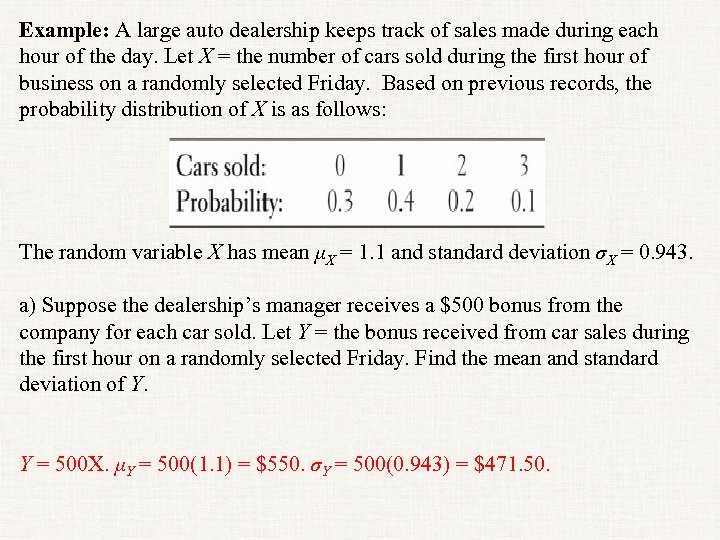Example: A large auto dealership keeps track of sales made during each hour of the day. Let X = the number of cars sold during the first hour of business on a randomly selected Friday. Based on previous records, the probability distribution of X is as follows: The random variable X has mean μX = 1. 1 and standard deviation σX = 0. 943. a) Suppose the dealership’s manager receives a \$500 bonus from the company for each car sold. Let Y = the bonus received from car sales during the first hour on a randomly selected Friday. Find the mean and standard deviation of Y. Y = 500 X. μY = 500(1. 1) = \$550. σY = 500(0. 943) = \$471. 50.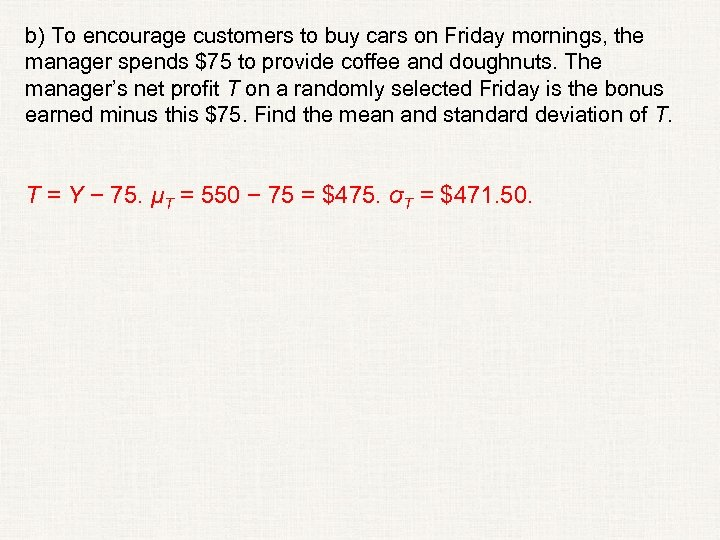b) To encourage customers to buy cars on Friday mornings, the manager spends \$75 to provide coffee and doughnuts. The manager’s net profit T on a randomly selected Friday is the bonus earned minus this \$75. Find the mean and standard deviation of T. T = Y − 75. μT = 550 − 75 = \$475. σT = \$471. 50.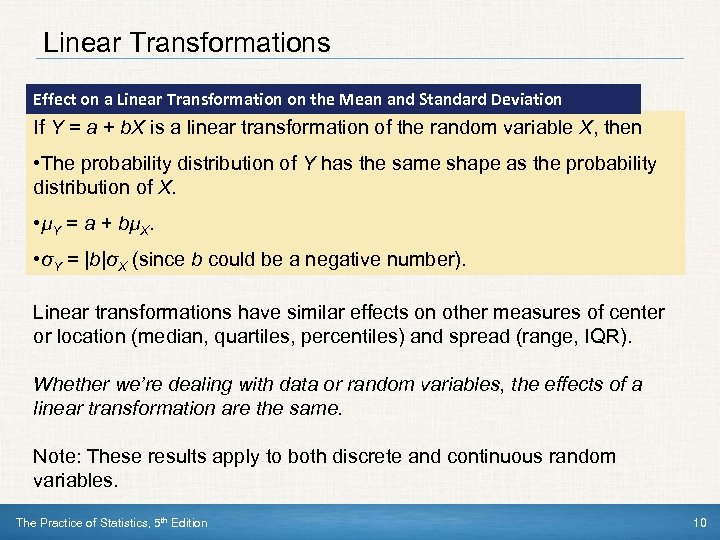Linear Transformations Effect on a Linear Transformation on the Mean and Standard Deviation If Y = a + b. X is a linear transformation of the random variable X, then • The probability distribution of Y has the same shape as the probability distribution of X. • µY = a + bµX. • σY = |b|σX (since b could be a negative number). Linear transformations have similar effects on other measures of center or location (median, quartiles, percentiles) and spread (range, IQR). Whether we’re dealing with data or random variables, the effects of a linear transformation are the same. Note: These results apply to both discrete and continuous random variables. The Practice of Statistics, 5 th Edition 10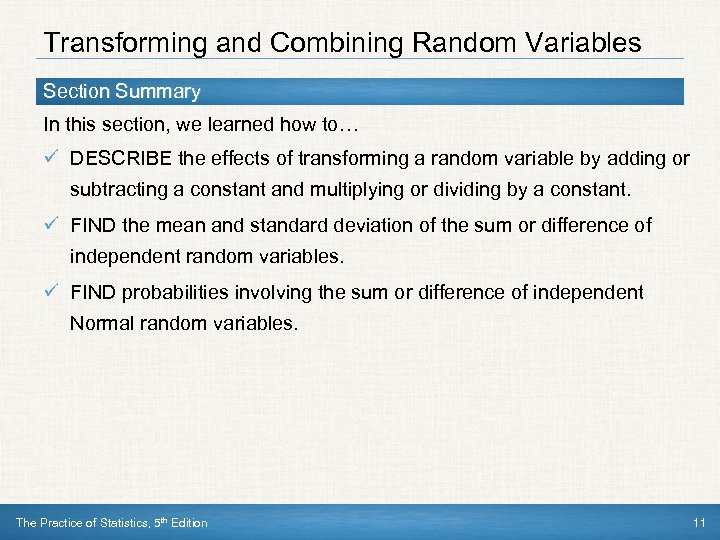Transforming and Combining Random Variables Section Summary In this section, we learned how to… ü DESCRIBE the effects of transforming a random variable by adding or subtracting a constant and multiplying or dividing by a constant. ü FIND the mean and standard deviation of the sum or difference of independent random variables. ü FIND probabilities involving the sum or difference of independent Normal random variables. The Practice of Statistics, 5 th Edition 11